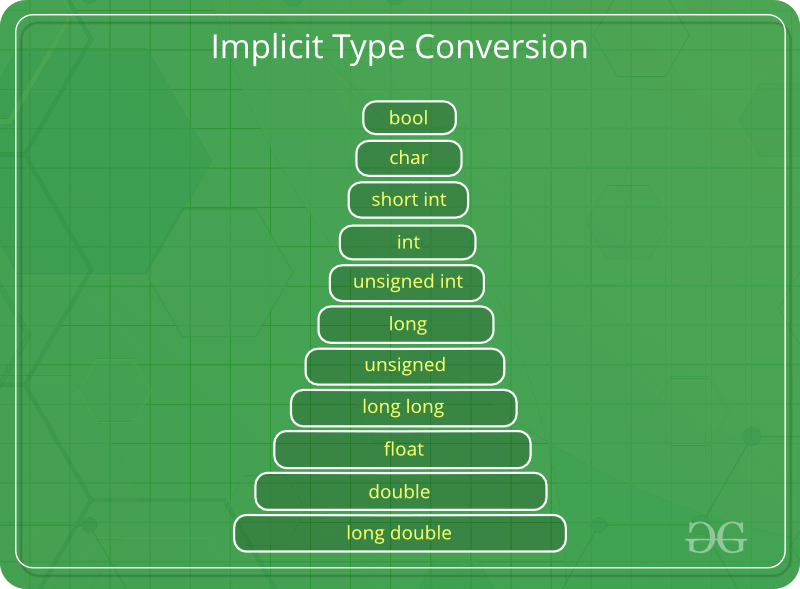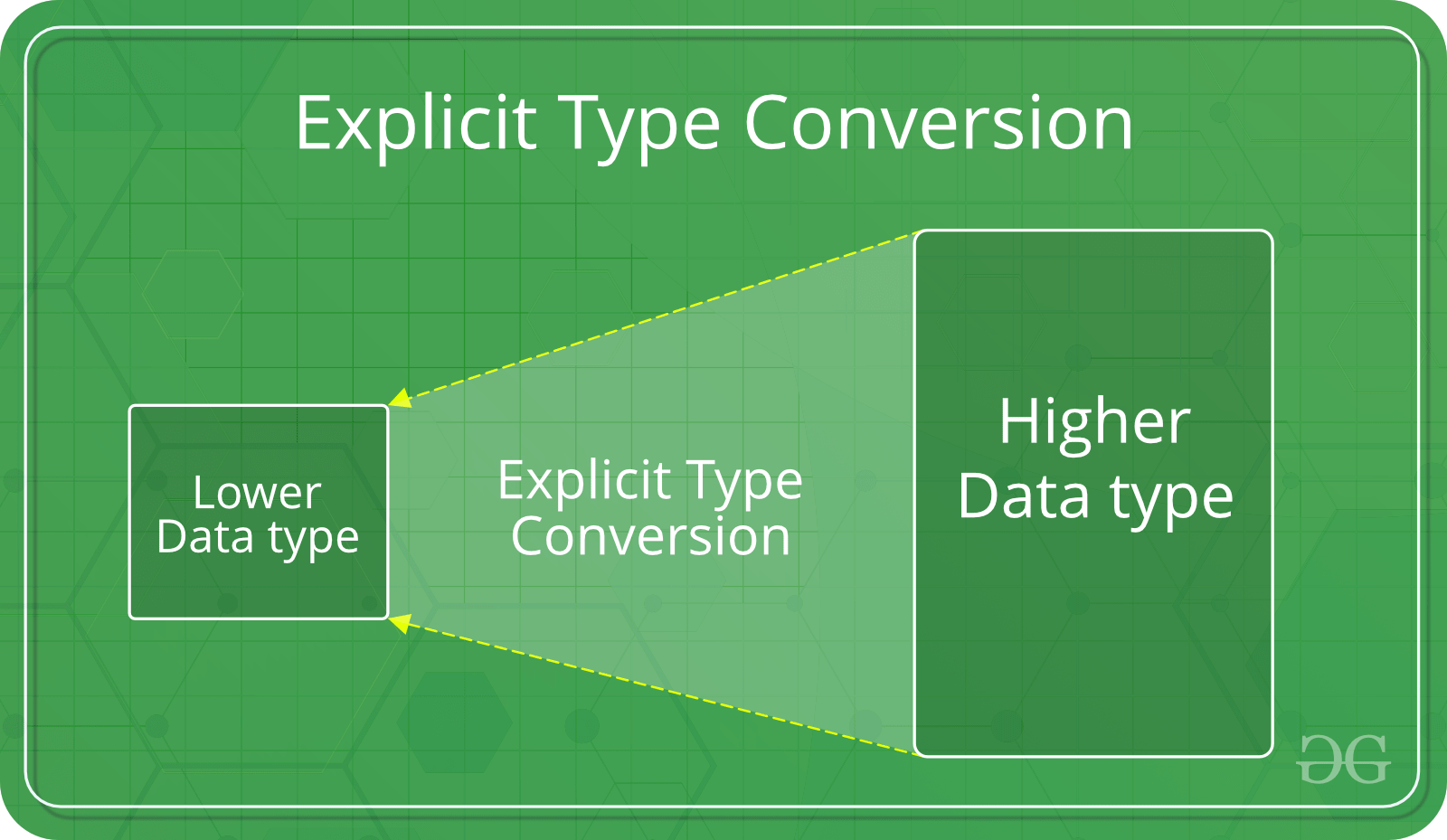Type Conversion in C

• Difficulty Level : Easy
• Last Updated : 25 Nov, 2020

A type cast is basically a conversion from one type to another. There are two types of type conversion:

1. Implicit Type ConversionAlso known as ‘automatic type conversion’.

• Done by the compiler on its own, without any external trigger from the user.
• Generally takes place when in an expression more than one data type is present. In such condition type conversion (type promotion) takes place to avoid loss of data.
• All the data types of the variables are upgraded to the data type of the variable with largest data type.

bool -> char -> short int -> int ->
unsigned int -> long -> unsigned ->
long long -> float -> double -> long double

• It is possible for implicit conversions to lose information, signs can be lost (when signed is implicitly converted to unsigned), and overflow can occur (when long long is implicitly converted to float).

Example of Type Implicit Conversion:

 // An example of implicit conversion#includeint main(){    int x = 10;    // integer x    char y = 'a';  // character c      // y implicitly converted to int. ASCII     // value of 'a' is 97    x = x + y;         // x is implicitly converted to float    float z = x + 1.0;      printf("x = %d, z = %f", x, z);    return 0;}

Output:

x = 107, z = 108.000000
1. Explicit Type ConversionThis process is also called type casting and it is user defined. Here the user can type cast the result to make it of a particular data type.

The syntax in C:

(type) expression

Type indicated the data type to which the final result is converted.

 // C program to demonstrate explicit type casting#include  int main(){    double x = 1.2;      // Explicit conversion from double to int    int sum = (int)x + 1;      printf("sum = %d", sum);      return 0;}

Output:

sum = 2